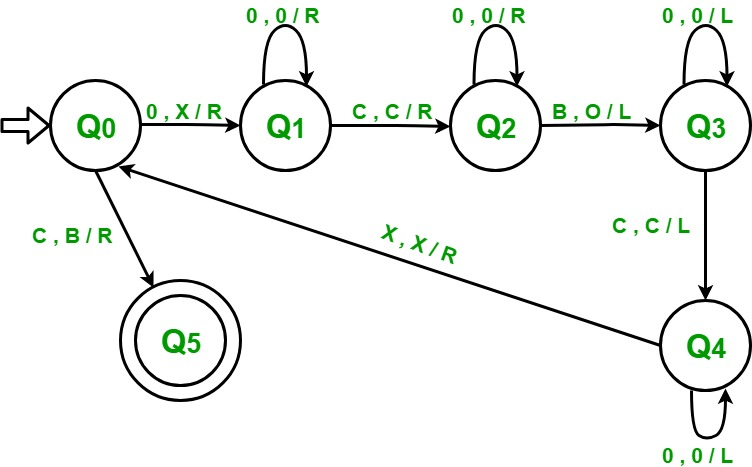GeeksforGeeks App
Open AppBrowser
Continue

## Related Articles

Prerequisite – Turing Machine
A number is represented in binary format in different finite automata. For example, 5 is represented as 101. However, in the case of addition using a Turing machine, unary format is followed. In unary format, a number is represented by either all ones or all zeroes. For example, 5 will be represented by a sequence of five zeroes or five ones: 5 = 1 1 1 1 1 or 5 = 0 0 0 0 0. Lets use zeroes for representation.

A Turing machine can be designed to perform addition by using its tape to represent the numbers to be added and its states to control the addition process. Here’s a high-level description of the process:

1. The two numbers to be added are placed on the tape, separated by a blank symbol.
2. The Turing machine starts at the leftmost digit of the first number and moves to the right, one digit at a time, checking the value of each digit.
3. If a digit is 0, the machine writes a 0 in the corresponding position of the sum. If it’s 1, it writes a 1.
4. If both digits are 1, the machine writes a 0 and adds a carry of 1 to the next position.
5. When the Turing machine reaches the end of the input, it adds any remaining carry and writes the result on the tape.

For adding 2 numbers using a Turing machine, both these numbers are given as input to the Turing machine separated by a “c”.

Examples – (2 + 3) will be given as 0 0 c 0 0 0:

```Input  :  0 0 c 0 0 0    // 2 + 3
Output :  0 0 0 0 0      // 5

Input  :  0 0 0 0 c 0 0 0  // 4 + 3
Output :  0 0 0 0 0 0 0    // 7```

Approach used –
Convert a 0 in the first number in to X and then traverse entire input and convert the first blank encountered into 0. Then move towards left ignoring all 0’s and “c”. Come the position just next to X, and repeat the same procedure until the time we get a “c” instead of X on returning. Convert the c into blank and addition is completed.

Steps –

Step-1: Convert 0 into X and go to step 2. If symbol is “c” then convert it into blank(B), move right and go to step 6.

Step-2: Keep ignoring 0’s and move towards right. Ignore “c”, move right and go to step 3.

Step-3: Keep ignoring 0’s and move towards right. Convert a blank (B) into 0, move left and go to step 4.

Step-4: Keep ignoring 0’s and move towards left. Ignore “c”, move left and go to step 3.

Step-5: Keep ignoring 0’s and move towards left. Ignore an X, move right and go to step 1.

Step-6: End.My Personal Notes arrow_drop_up